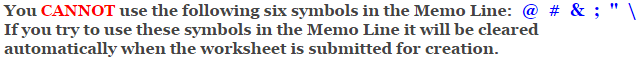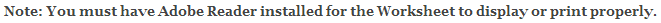# Fractions Worksheets

## Multiplying Mixed Numbers Worksheets

This fraction worksheet is great for practicing Multiplying Mixed Numbers Problems. The problems may be selected for two different degrees of difficulty. The easiest will keep the denominators 2, 3, 4, 5, & 10 and the numerators between 1 and 9. The hardest will keep the denominators 2 thru 10 and the numerators between 1 and 9. The whole numbers may be 2, 3, or 4. The answer worksheet will show the progression on how to solve the problems. First rewrite the problem and combine the whole numbers into the fractions, next multiply the numerators and then the denominators. Then check to see if we need to simplify or reduce the fraction. This fraction worksheet will generate 10 or 15 fraction mixed number multiplication problems per worksheet, and remember every time you create a worksheet the problems will change and will not repeat.

### Difficulty

Numerators 1 thru 9.
Denominators 2, 3, 4, 5, & 10.

Numerators 1 thru 9.
Denominators 2 thru 10.

### Language for the Fractions Worksheet

 English German Albanian Spanish Swedish Italian French Turkish Polish Norwegian

### Memo Line for the Fractions Worksheet

You may enter a message or special instruction that will appear on the bottom left corner of the Fractions Worksheet.Now you are ready to create your Fractions Worksheet by pressing the Create Button.

 Recommended Videos

If You Experience Display Problems with Your Math Worksheet